Скачать презентацию J. M. Gabrielse. Ray Diagrams. Images, Mirrors and

ray_diagrams_images_mirrors_lenses.ppt

• Размер: 980.5 Кб
• Количество слайдов: 54

## Описание презентации J. M. Gabrielse. Ray Diagrams. Images, Mirrors and по слайдамJ. M. Gabrielse. Ray Diagrams. Images, Mirrors and Lenses Adapted from J. M. Gabreilse For Grade 11 PhysicsJ. M. Gabrielse. Objectives : • To draw ray diagrams • To see how real and virtual images are formed • To use different object distances and focal lengths to create different sized images • To gain a working knowledge of the terms: real image , virtual image , upright , inverted , magnified and diminished.J. M. Gabrielse. Real or Virtual Images • Real Images are formed when light ray do come together to form an image. • Virtual images are formed when light rays seem to come together to form an image. • Sight lines are extensions of light rays needed to show the perceived virtual image. • Sight lines are dashed lines in ray diagrams.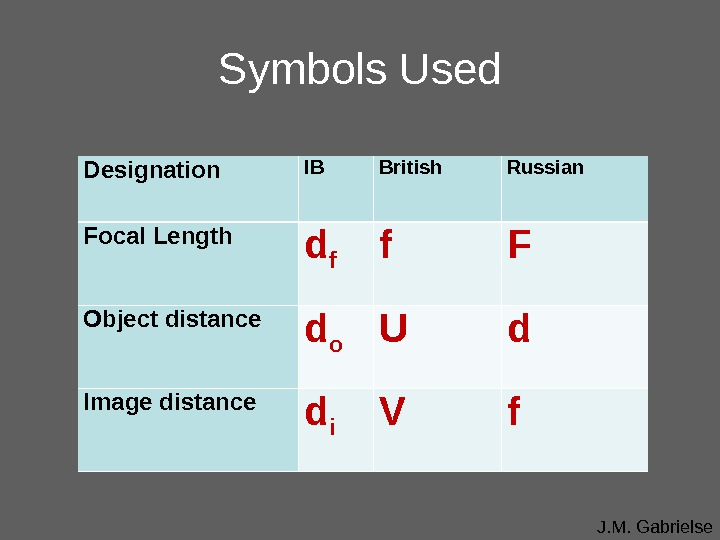J. M. Gabrielse. Symbols Used Designation IB British Russian Focal Length d f f F Object distance d o U d Image distance d i V f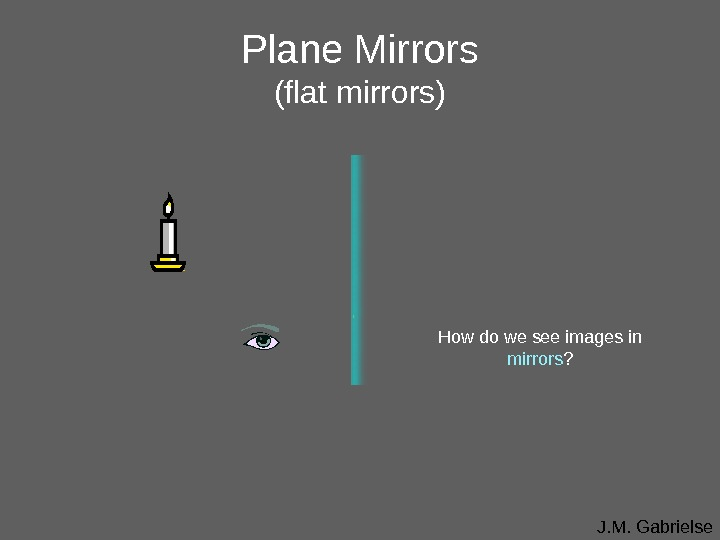J. M. Gabrielse. Plane Mirrors (flat mirrors) How do we see images in mirrors ?J. M. Gabrielse. Plane Mirrors (flat mirrors) object image Light reflected off the mirror converges to form an image in the eye. How do we see images in mirrors ?J. M. Gabrielse. Plane Mirrors (flat mirrors) object image Light reflected off the mirror converges to form an image in the eye. The eye perceives light rays as if they came through the mirror. Imaginary light rays extended behind mirrors are called sight lines. How do we see images in mirrors ?J. M. Gabrielse. Plane Mirrors (flat mirrors) object image Light reflected off the mirror converges to form an image in the eye. The eye perceives light rays as if they came through the mirror. Imaginary light rays extended behind mirrors are called sight lines. Image is virtual since it is formed by imaginary sight lines, not real light rays. How do we see images in mirrors ?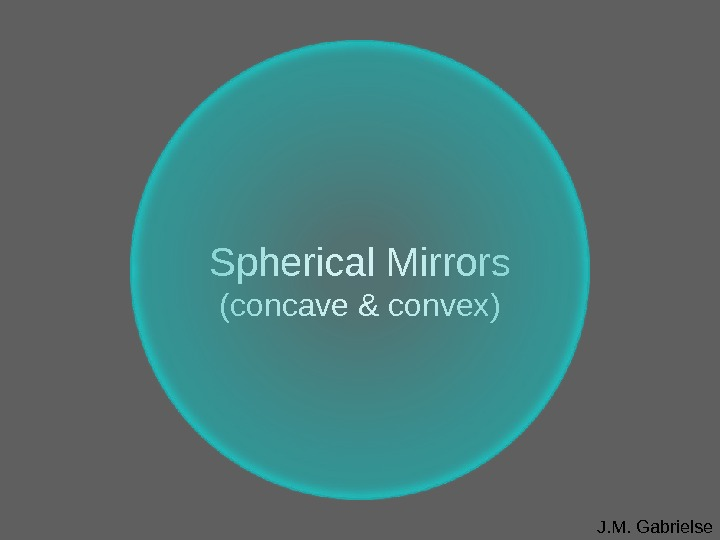J. M. Gabrielse. Spherical Mirrors (concave & convex)J. M. Gabrielse. Concave & Convex Mirrors are part of a sphere C: the center point of the sphere r: radius of curvature = the radius of the sphere F: focal point is halfway between C and the mirror f: the focal distance, f = r/2 r f • C •J. M. Gabrielseoptical axis. Concave Mirrors (caved in) • F Light rays that come in parallel to the optical axis reflect through the focal point.J. M. Gabrielseoptical axis. Concave Mirror (Object distance: d o > d f ) •J. M. Gabrielseoptical axis. Concave Mirror (Object distance: d o > d f ) • F The first ray comes in parallel to the optical axis and reflects through the focal point.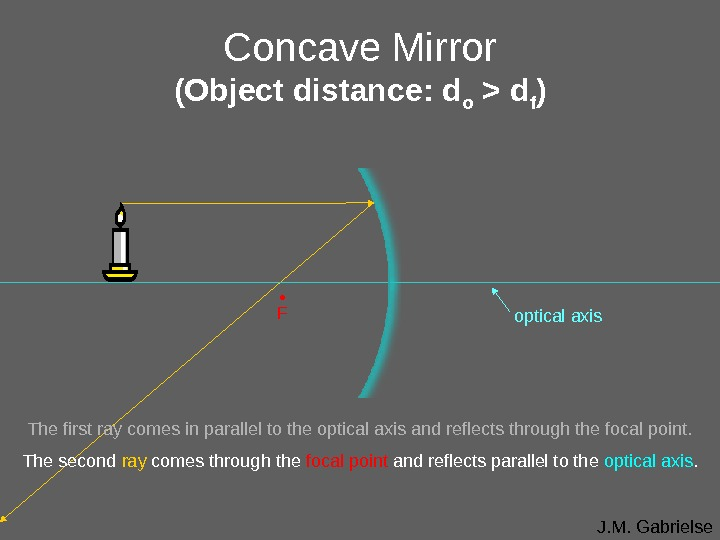J. M. Gabrielseoptical axis. Concave Mirror (Object distance: d o > d f ) • F The first ray comes in parallel to the optical axis and reflects through the focal point. The second ray comes through the focal point and reflects parallel to the optical axis.J. M. Gabrielseoptical axis. Concave Mirror (Object distance: d o > d f ) • F The first ray comes in parallel to the optical axis and reflects through the focal point. The second ray comes through the focal point and reflects parallel to the optical axis. A real, inverted, diminished image forms where the light rays converge.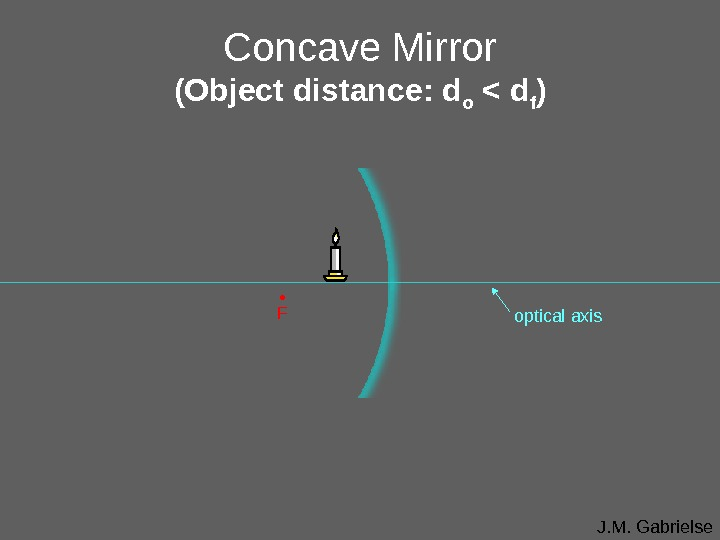J. M. Gabrielseoptical axis. Concave Mirror (Object distance: d o < d f ) •J. M. Gabrielseoptical axis. Concave Mirror (Object distance: d o < d f ) • F The first ray comes in parallel to the optical axis and reflects through the focal point.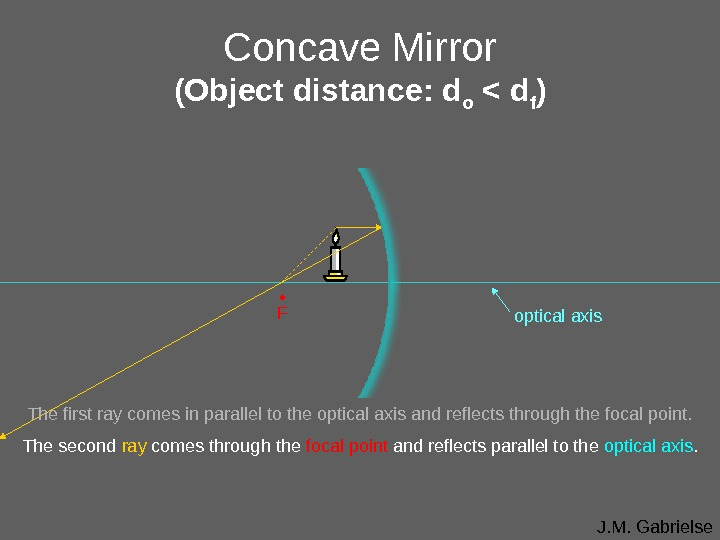J. M. Gabrielseoptical axis. Concave Mirror (Object distance: d o < d f ) • F The first ray comes in parallel to the optical axis and reflects through the focal point. The second ray comes through the focal point and reflects parallel to the optical axis.J. M. Gabrielseoptical axis. Concave Mirror (Object distance: d o < d f ) • F The first ray comes in parallel to the optical axis and reflects through the focal point. The second ray comes through the focal point and reflects parallel to the optical axis. The image forms where the rays converge. But they don’t seem to converge.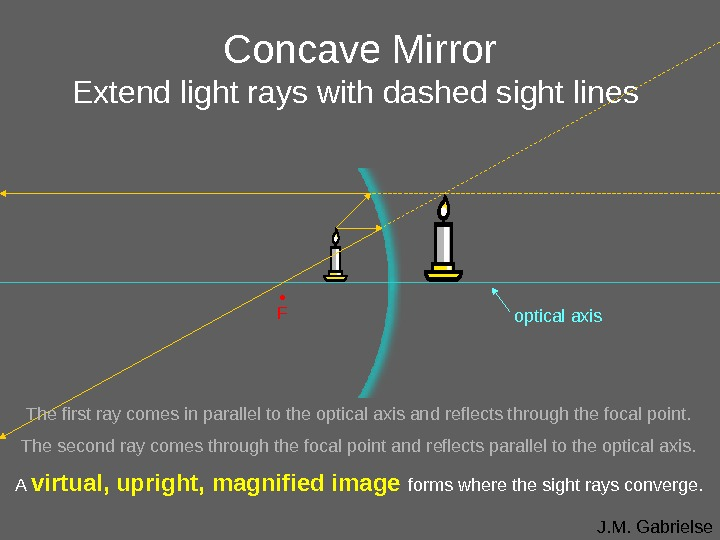J. M. Gabrielseoptical axis. Concave Mirror Extend light rays with dashed sight lines • F The first ray comes in parallel to the optical axis and reflects through the focal point. The second ray comes through the focal point and reflects parallel to the optical axis. A virtual, upright, magnified image forms where the sight rays converge.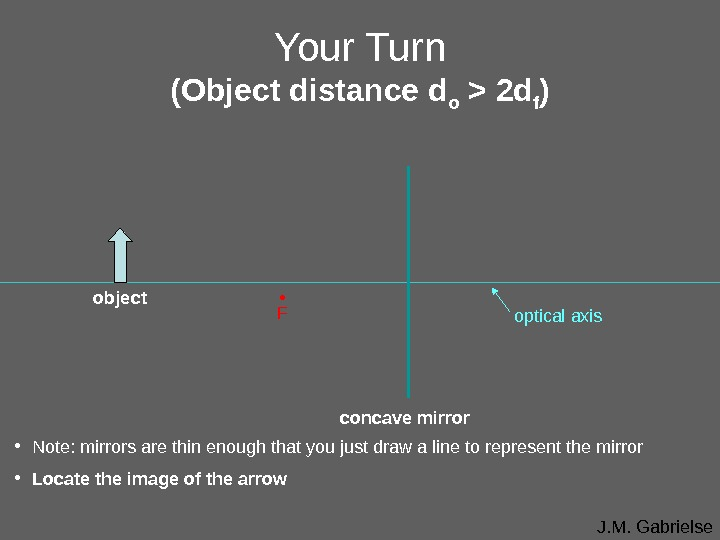J. M. Gabrielseoptical axis. Your Turn (Object distance d o > 2 d f ) • Fobject concave mirror • Note: mirrors are thin enough that you just draw a line to represent the mirror • Locate the image of the arrow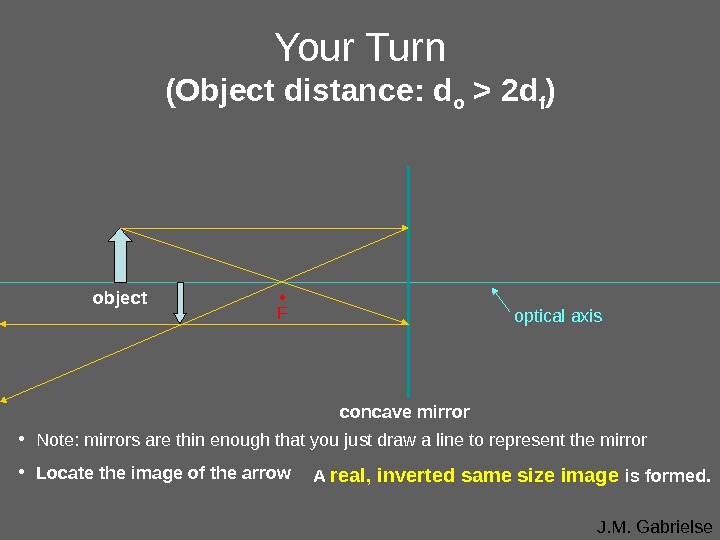J. M. Gabrielseoptical axis. Your Turn (Object distance: d o > 2 d f ) • Fobject concave mirror • Note: mirrors are thin enough that you just draw a line to represent the mirror • Locate the image of the arrow A real, inverted same size image is formed.J. M. Gabrielse. Convex Mirrors (curved out) Light rays that come in parallel to the optical axis reflect from the focal point. optical axis • F The focal point is considered virtual since sight lines, not light rays , go through it.J. M. Gabrielse. Convex Mirror (Object distance d o > 2 d f ) optical axis •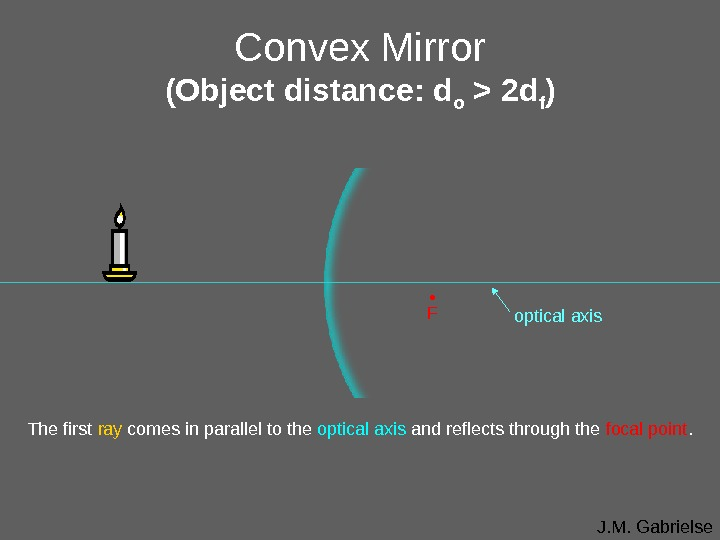J. M. Gabrielse. Convex Mirror (Object distance: d o > 2 d f ) optical axis • F The first ray comes in parallel to the optical axis and reflects through the focal point.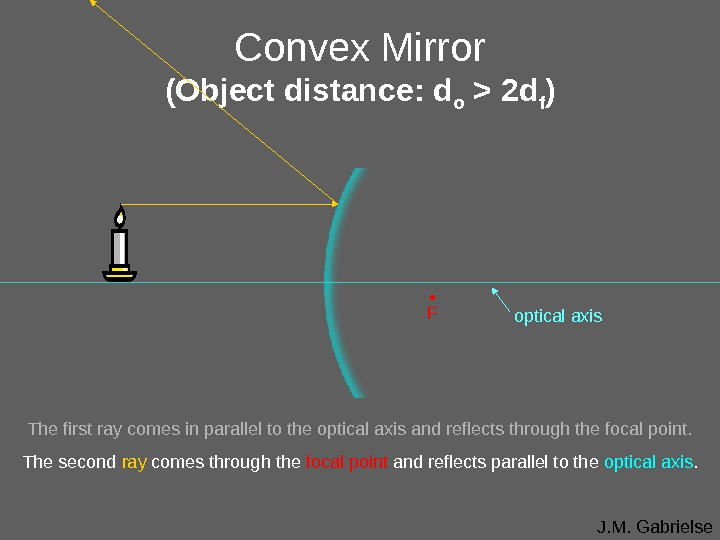J. M. Gabrielse. Convex Mirror (Object distance: d o > 2 d f ) optical axis • F The first ray comes in parallel to the optical axis and reflects through the focal point. The second ray comes through the focal point and reflects parallel to the optical axis.J. M. Gabrielse. Convex Mirror (Object distance: d o > 2 d f ) optical axis • F The first ray comes in parallel to the optical axis and reflects through the focal point. The second ray comes through the focal point and reflects parallel to the optical axis. The light rays don’t converge, but the sight lines do.J. M. Gabrielse. Convex Mirror (Object distance: d o > 2 d f ) optical axis • F The first ray comes in parallel to the optical axis and reflects through the focal point. The second ray comes through the focal point and reflects parallel to the optical axis. The light rays don’t converge, but the sight lines do. A virtual, upright, diminished image forms where the sight lines converge.J. M. Gabrielse optical axis. Your Turn (Convex Mirror) • F • Note: mirrors are thin enough that you just draw a line to represent the mirror • Locate the image of the arrow object convex mirrorJ. M. Gabrielse optical axis. Your Turn (Convex Mirror) • F • Note: mirrors are thin enough that you just draw a line to represent the mirror • Locate the image of the arrow object convex mirror image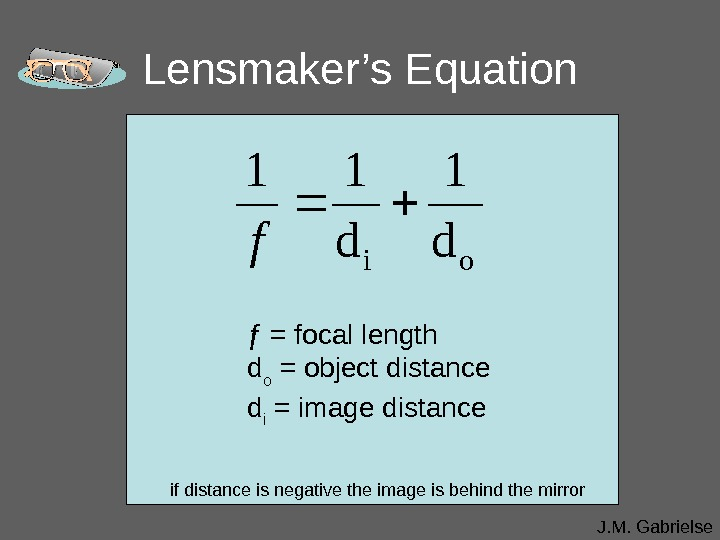J. M. Gabrielse. Lensmaker’s Equation ƒ = focal length d o = object distance d i = image distanceoi d 11 f if distance is negative the image is behind the mirrorJ. M. Gabrielse. Magnification Equationo i d d h h m m = magnification h i = image height h o = object height If height is negative the image is upside down if the magnification is negative the image is inverted (upside down)J. M. Gabrielse. Refraction (bending light ) Refraction is when light bends as it passes from one medium into another. When light traveling through air passes into the glass block it is refracted towards the normal. When light passes back out of the glass into the air , it is refracted away from the normal. Since light refracts when it changes mediums it can be aimed. Lenses are shaped so light is aimed at a focal point. normalair airθ rθ i glass blockJ. M. Gabrielse. Lenses The first telescope, designed and built by Galileo, used lenses to focus light from faraway objects, into Galileo’s eye. His telescope consisted of a concave lens and a convex lens. Light rays are always refracted (bent) towards the thickest part of the lens. convex lens concave lenslight from object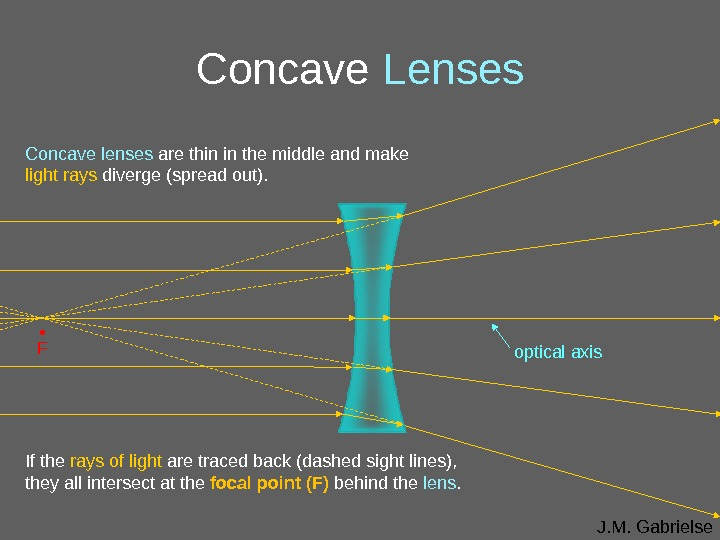J. M. Gabrielse. Concave Lenses Concave lenses are thin in the middle and make light rays diverge (spread out). If the rays of light are traced back (dashed sight lines), they all intersect at the focal point (F) behind the lens. optical axis •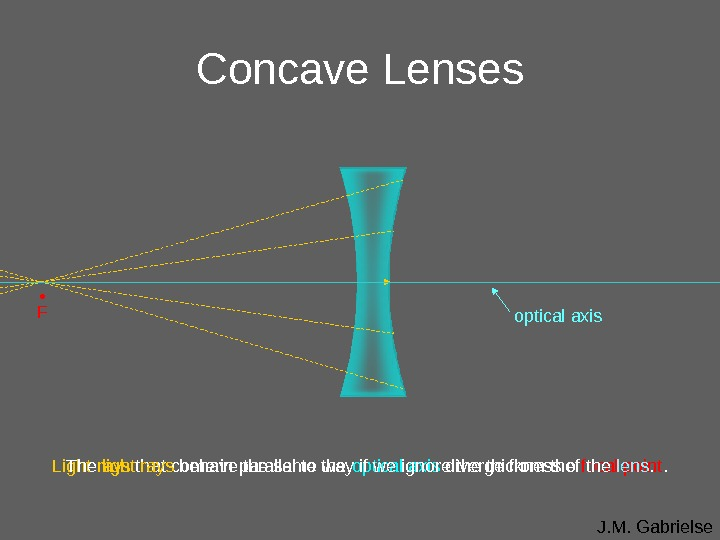J. M. Gabrielse • F optical axis Light rays that come in parallel to the optical axis diverge from the focal point. Concave Lenses The light rays behave the same way if we ignore thickness of the lens.J. M. Gabrielse. Concave Lenses optical axis • F Light rays that come in parallel to the optical axis still diverge from the focal point.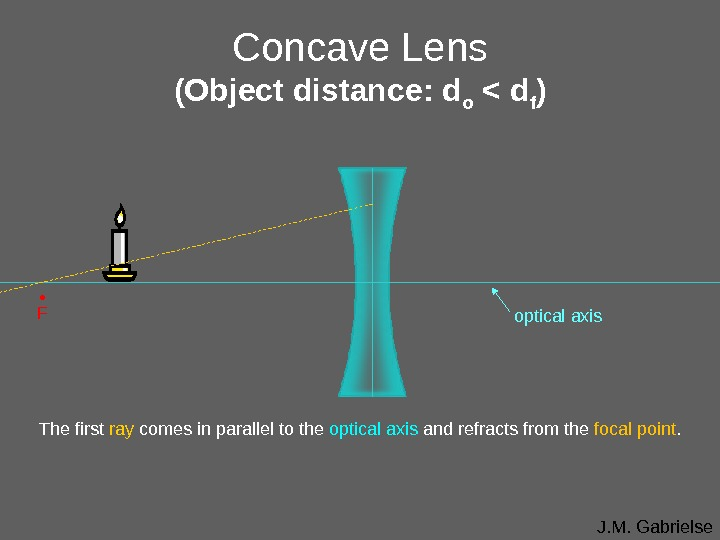J. M. Gabrielse. Concave Lens (Object distance: d o < d f ) The first ray comes in parallel to the optical axis and refracts from the focal point. optical axis •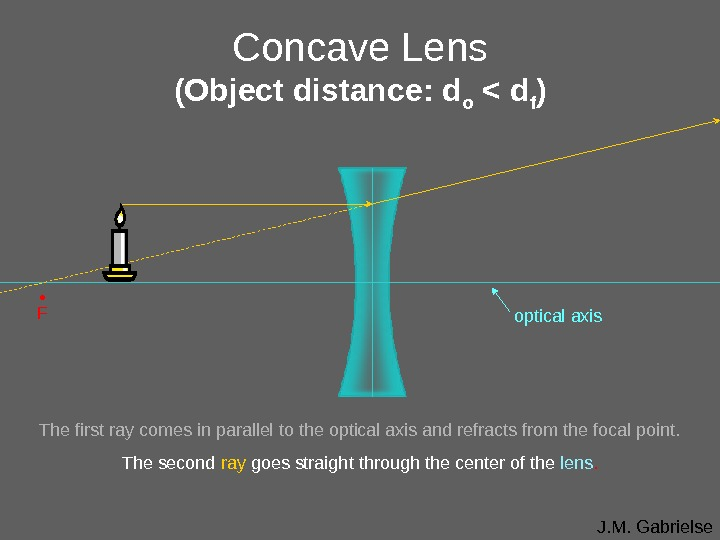J. M. Gabrielse. Concave Lens (Object distance: d o < d f ) optical axis • F The first ray comes in parallel to the optical axis and refracts from the focal point. The second ray goes straight through the center of the lens.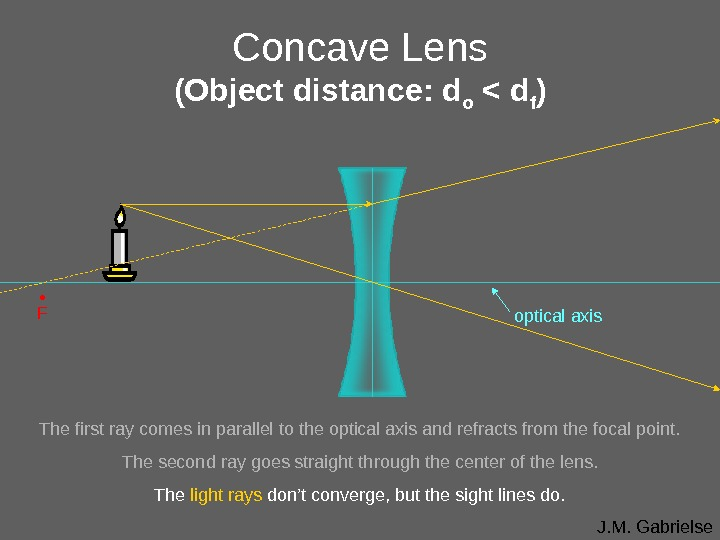J. M. Gabrielse. Concave Lens (Object distance: d o < d f ) optical axis • F The first ray comes in parallel to the optical axis and refracts from the focal point. The second ray goes straight through the center of the lens. The light rays don’t converge, but the sight lines do.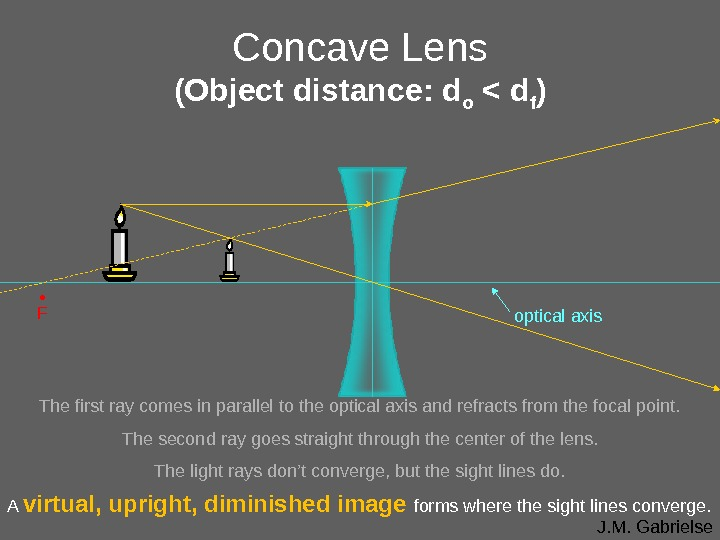J. M. Gabrielse. Concave Lens (Object distance: d o < d f ) optical axis • F The first ray comes in parallel to the optical axis and refracts from the focal point. The second ray goes straight through the center of the lens. The light rays don’t converge, but the sight lines do. A virtual, upright, diminished image forms where the sight lines converge.J. M. Gabrielse optical axis. Your Turn (Object distance: d o > 2 d f ) • F • Note: lenses are thin enough that you just draw a line to represent the lens. • Locate the image of the arrow. object concave lens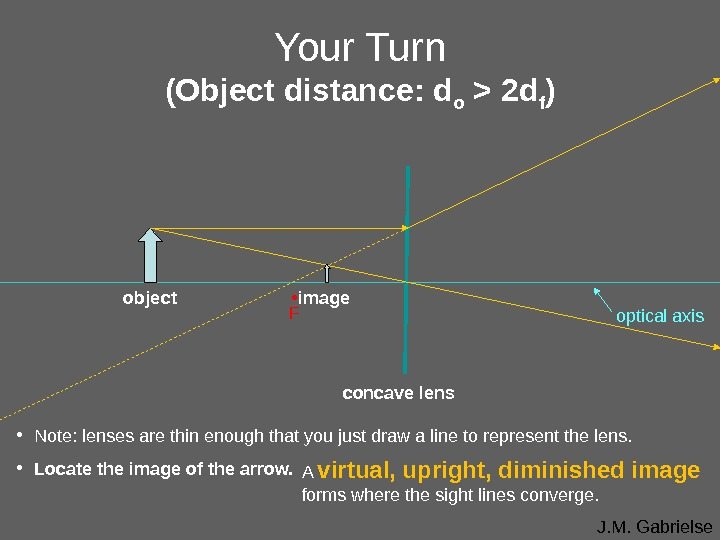J. M. Gabrielse optical axis. Your Turn (Object distance: d o > 2 d f ) • F • Note: lenses are thin enough that you just draw a line to represent the lens. • Locate the image of the arrow. object concave lensimage A virtual, upright, diminished image forms where the sight lines converge.J. M. Gabrielse. Convex Lenses Convex lenses are thicker in the middle and focus light rays to a focal point in front of the lens. The focal length of the lens is the distance between the center of the lens and the point where the light rays are focused.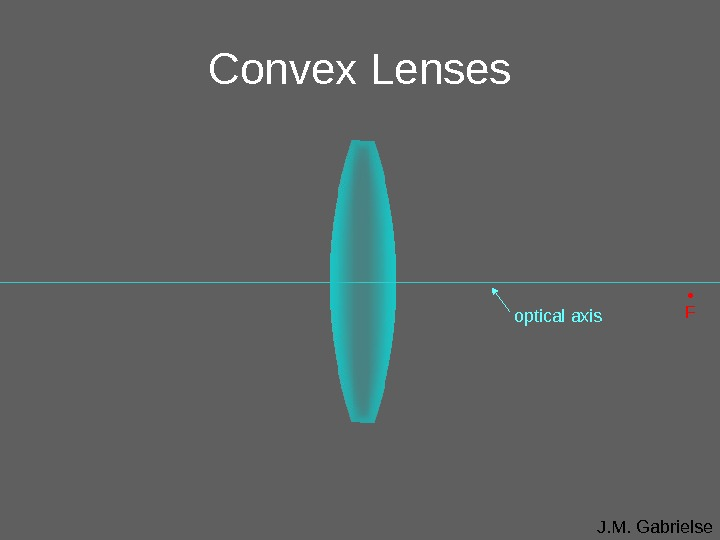J. M. Gabrielse. Convex Lenses optical axis •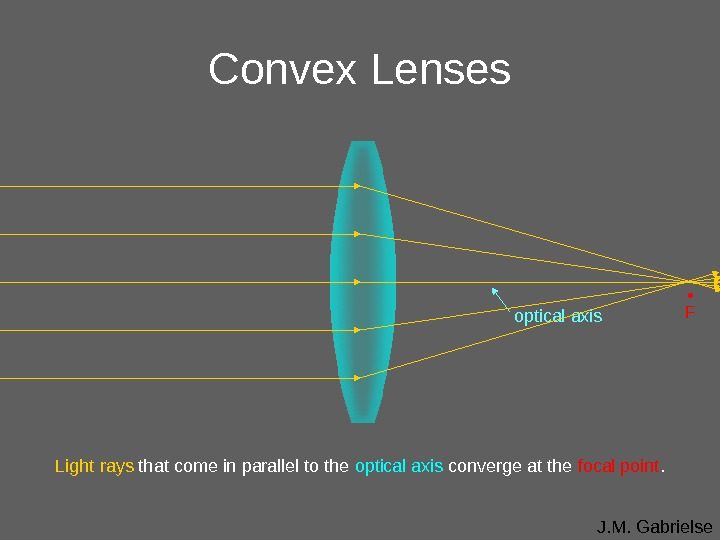J. M. Gabrielse. Convex Lenses optical axis Light rays that come in parallel to the optical axis converge at the focal point. •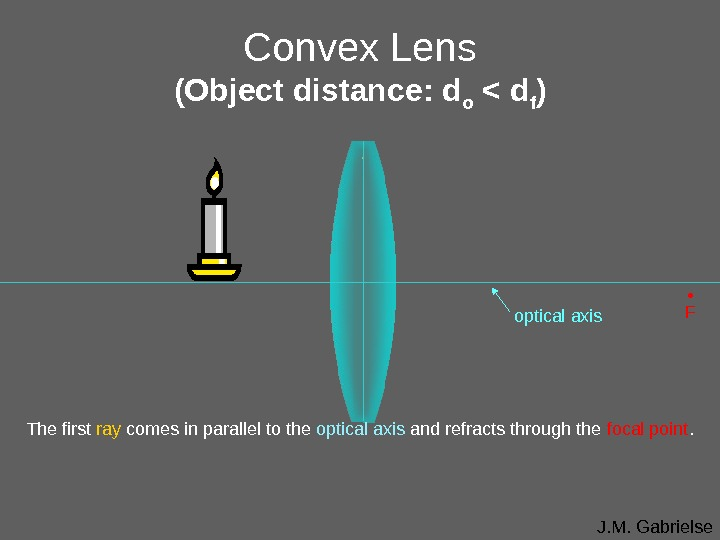J. M. Gabrielse. Convex Lens (Object distance: d o < d f ) • F The first ray comes in parallel to the optical axis and refracts through the focal point. optical axis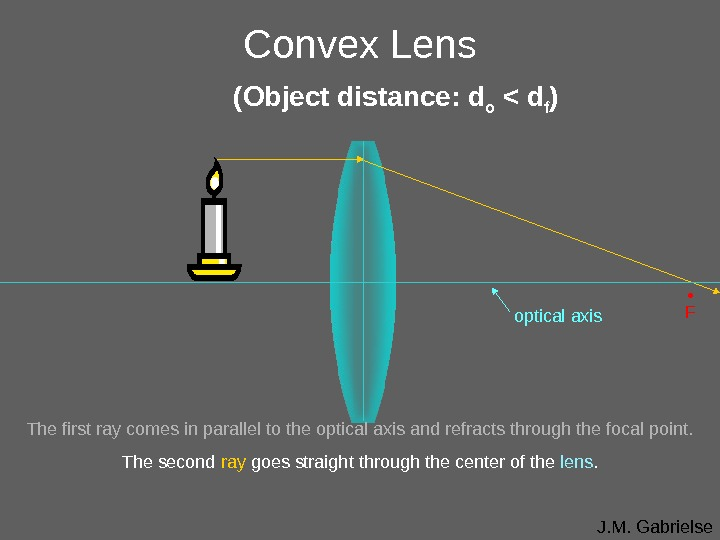J. M. Gabrielse. Convex Lens (Object distance: d o < d f ) • F The first ray comes in parallel to the optical axis and refracts through the focal point. The second ray goes straight through the center of the lens. optical axis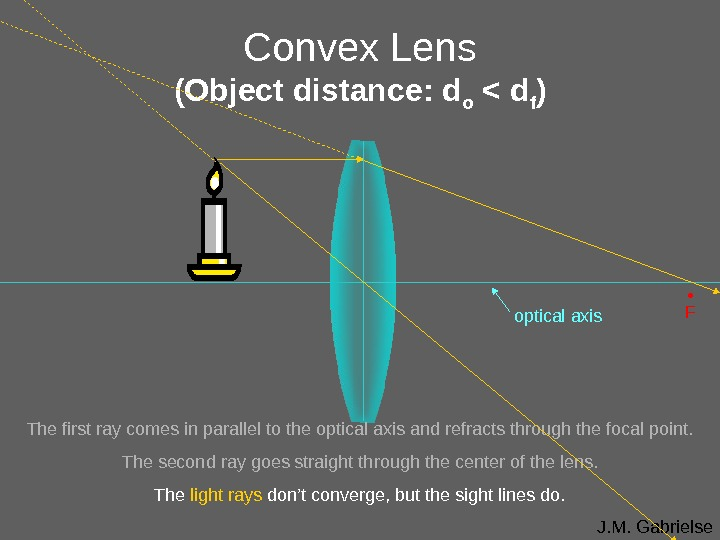J. M. Gabrielse. Convex Lens (Object distance: d o < d f ) optical axis • F The first ray comes in parallel to the optical axis and refracts through the focal point. The second ray goes straight through the center of the lens. The light rays don’t converge, but the sight lines do.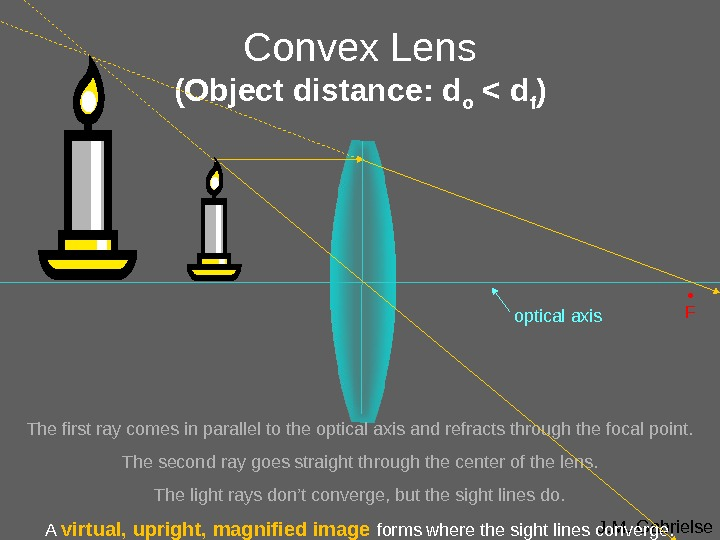J. M. Gabrielse. Convex Lens (Object distance: d o < d f ) optical axis • F The first ray comes in parallel to the optical axis and refracts through the focal point. The second ray goes straight through the center of the lens. The light rays don’t converge, but the sight lines do. A virtual, upright, magnified image forms where the sight lines converge.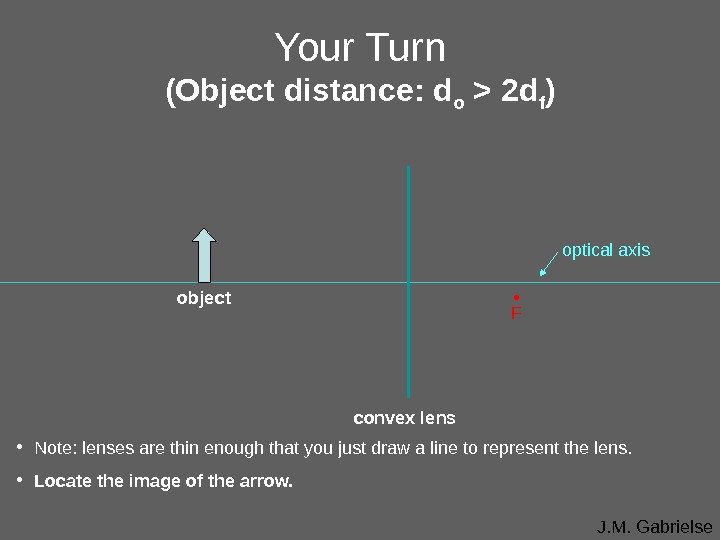J. M. Gabrielseoptical axis. Your Turn (Object distance: d o > 2 d f ) • F • Note: lenses are thin enough that you just draw a line to represent the lens. • Locate the image of the arrow. object convex lens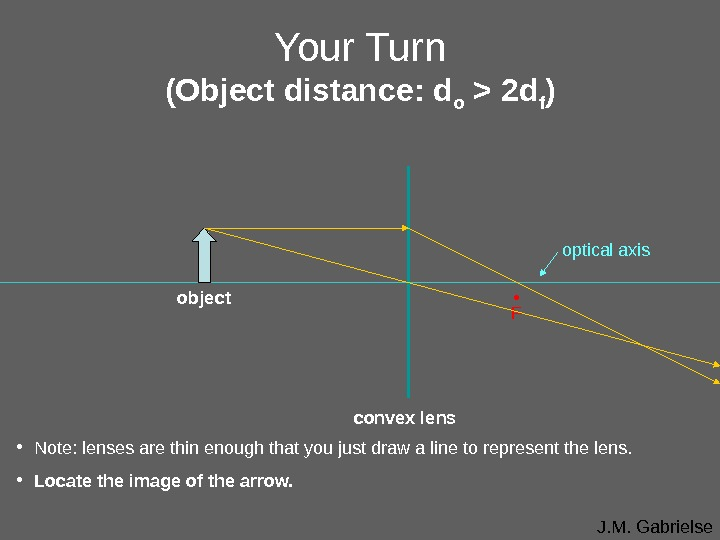J. M. Gabrielseoptical axis. Your Turn (Object distance: d o > 2 d f ) • F • Note: lenses are thin enough that you just draw a line to represent the lens. • Locate the image of the arrow. object convex lensJ. M. Gabrielseoptical axis. Your Turn (Object distance: d o = 2 d f ) • F • Note: lenses are thin enough that you just draw a line to represent the lens. • Locate the image of the arrow. object convex lens image A real, inverted, same size image forms where the light rays converge.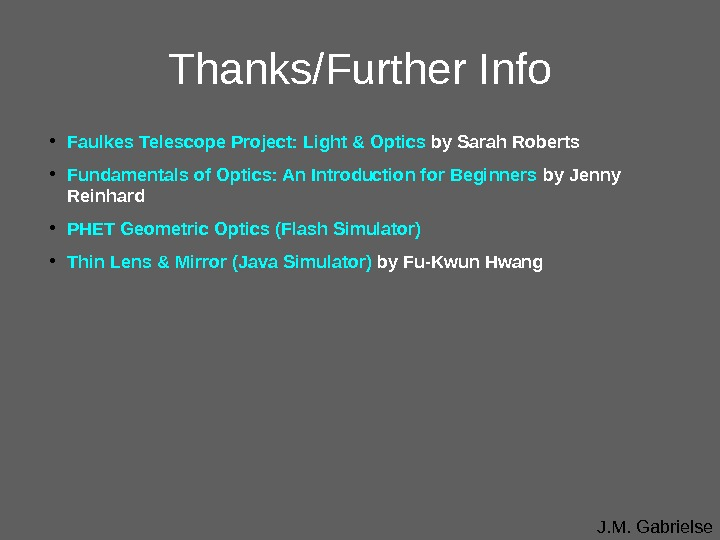J. M. Gabrielse. Thanks/Further Info • Faulkes Telescope Project: Light & Optics by Sarah Roberts • Fundamentals of Optics: An Introduction for Beginners by Jenny Reinhard • PHET Geometric Optics (Flash Simulator) • Thin Lens & Mirror (Java Simulator) by Fu-Kwun Hwang